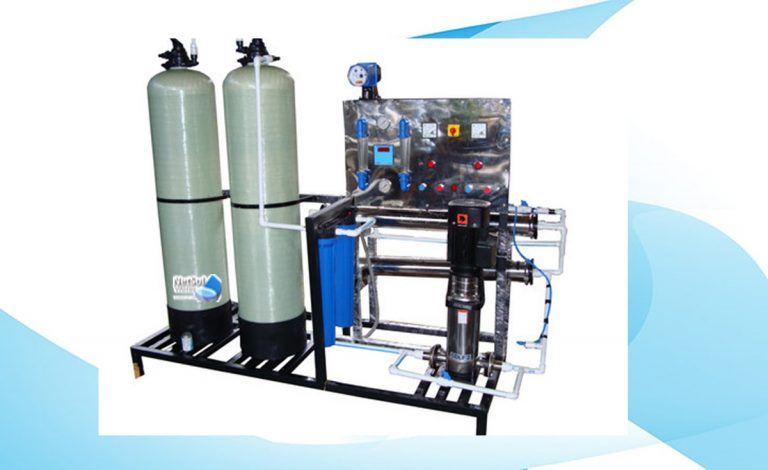# Cost of treated water in RO Plants## How to analyze the cost per litre of treated water in RO Plants?

A – For 2000 LPH supply of Cost of treated water in RO Plants system

Approximate cost of unit: Rs. 7,05,000/-

Electricity Requirement: 6.25 KW = 6.25 x 16 = 100 KW

Note: 1- Assuming 16Hrs. working daily.

2- Power consumption by the equipment is taken as provided by manufacturer.

Electricity charges at Rs.8.36/ KW for 365 days = 100×8.36×365 = Rs.3,05,140/-

Labour AMC cost/ year: 10,000/-

Consumables (Assuming normal service requirement): Rs. 2,15,890/

Note: Assuming life of RO system as 5 Years, Annual cost of one unit – Rs.1,41,000.00/

Total cost involved/ year = Rs. 1,41,000.00 + Rs. 3,05,140.00+ Rs. 10,000.00 + Rs. 2,15,890.00

= Rs. 6,72,030.00/

B – For 1000 LPH supply Commercial RO Plant

Approximate cost of unit: Rs. 5,80,000/-

Electricity Requirement:  4.25 KW = 4.25 x 16 = 68 KW

Note: 1- Assuming 16Hrs. working daily.

2- Power consumption by the equipment is taken as provided by manufacturer.

Electricity charges at Rs.8.36 / KW for 365 days = 68x 8.36 x 365 = Rs.2,07,495/-

Labour AMC cost / year: 9,700/-

Consumables (Assuming normal service requirement):  Rs.127210/-

Note: Assuming life of RO system as 5 Years, annual cost of one unit – Rs.1,16,000.00/

Total cost involved / year= Rs. 1,16,000.00 + Rs. 2,07,495.00+ Rs. 9,7000.00 + Rs.127210.00

= Rs. 4,60,405.00/

C – For 500 LPH supply Commercial RO Plant

Approximate cost of unit: Rs. 2,75,000/-

Electricity Requirement: 3.25 KW = 3.25 x 16 = 52 KW

Note: 1- Assuming 16Hrs. working daily.

2- Power consumption by the equipment is taken as provided by manufacturer.

Electricity charges at Rs.8.36 / KW for 365 days = 52 x 8.36 x 365 = Rs.1,58,673/-

Labour AMC cost / year: 9,110/-

Consumables (Assuming normal service requirement): Rs.60,340/-

Note: Assuming life of RO system as 5 Years, annual cost of one unit – Rs.55,000.00/

Total cost involved / year= Rs. 55,000.00 + Rs. 1,58,673.00+ Rs. 9,110.00 + Rs. 60,340.00

= Rs. 2,83,123.00/

D – For 250 LPH supply Commercial RO Plant

Approximate cost of unit: Rs. 2,35,000/-

Electricity Requirement: 1.25 KW = 3.25 x 16 = 20 KW

Note: 1- Assuming 16Hrs. working daily.

2- Power consumption by the equipment is taken as provided by manufacturer.

Electricity charges at Rs.8.36/ KW for 365 days = 20 x 8.36 x 365 = Rs.61,028/-

Labour AMC cost / year: 9,110/-

Consumables (Assuming normal service requirement): Rs.30,350/-

Note: Assuming life of RO system as 5 Years, annual cost of one unit: Rs.47,000.00/

Total cost involved / year= Rs. 47,000.00 + Rs. 61,028.00+ Rs. 9,110.00 + Rs. 30,350.00

= Rs. 1,47,488.00

#### Schematic diagram of RO Plant

##### Conclusion

Typically, Commercial RO Plants from Netsol Water are created and operated as solitary machines that produce the necessary quantity throughout the entire day. These can be duplexed if necessary and a central control panel can be used to switch the RO plant on or off, manually or automatically. When there is a higher demand for water, the units can also run simultaneously, doubling the permeate production.

To learn more about our wide range of Commercial RO Plant manufacturers, call us at +91-9650608473 or send an email to enquiry@netsolwater.com